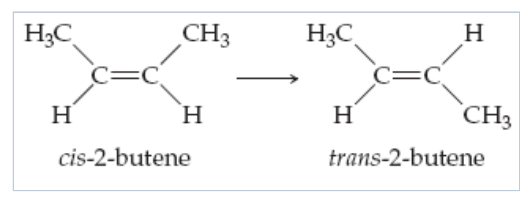# Problem: Part B. The average bond energy (enthalpy) for a C=C double bond is 614 kJ / mol and that of a C−C single bond is 348 kJ / mol. Estimate the energy needed to break only the π bond of the double bond of 2-butene.Express your answer numerically in joules per molecule.The molecule 2-butene, C4H8, can undergo a geometric change called cis-trans isomerization. Such transformations can be induced by light and are the key to human vision. Rotation about the C=C bond, which breaks the π bond, is required for the isomerization.

###### FREE Expert Solution
80% (314 ratings)View Complete Written Solution
###### Problem Details

Part B. The average bond energy (enthalpy) for a C=C double bond is 614 kJ / mol and that of a C−C single bond is 348 kJ / mol. Estimate the energy needed to break only the π bond of the double bond of 2-butene.